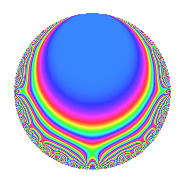Properties

 Label 29.2.dLevel 29 Weight 2 Character orbit d Rep. character $$\chi_{29}(7,\cdot)$$ Character field $$\Q(\zeta_{7})$$ Dimension 6 Newforms 1 Sturm bound 5 Trace bound 0

Related objects

Defining parameters

 Level: $$N$$ = $$29$$ Weight: $$k$$ = $$2$$ Character orbit: $$[\chi]$$ = 29.d (of order $$7$$ and degree $$6$$) Character conductor: $$\operatorname{cond}(\chi)$$ = $$29$$ Character field: $$\Q(\zeta_{7})$$ Newforms: $$1$$ Sturm bound: $$5$$ Trace bound: $$0$$

Dimensions

The following table gives the dimensions of various subspaces of $$M_{2}(29, [\chi])$$.

Total New Old
Modular forms 18 18 0
Cusp forms 6 6 0
Eisenstein series 12 12 0

Trace form

 $$6q$$ $$\mathstrut -\mathstrut 2q^{2}$$ $$\mathstrut -\mathstrut 5q^{3}$$ $$\mathstrut -\mathstrut 2q^{4}$$ $$\mathstrut +\mathstrut q^{5}$$ $$\mathstrut -\mathstrut 3q^{6}$$ $$\mathstrut +\mathstrut q^{7}$$ $$\mathstrut -\mathstrut 7q^{8}$$ $$\mathstrut +\mathstrut 6q^{9}$$ $$\mathstrut +\mathstrut O(q^{10})$$ $$6q$$ $$\mathstrut -\mathstrut 2q^{2}$$ $$\mathstrut -\mathstrut 5q^{3}$$ $$\mathstrut -\mathstrut 2q^{4}$$ $$\mathstrut +\mathstrut q^{5}$$ $$\mathstrut -\mathstrut 3q^{6}$$ $$\mathstrut +\mathstrut q^{7}$$ $$\mathstrut -\mathstrut 7q^{8}$$ $$\mathstrut +\mathstrut 6q^{9}$$ $$\mathstrut +\mathstrut 9q^{10}$$ $$\mathstrut -\mathstrut 11q^{11}$$ $$\mathstrut +\mathstrut 4q^{12}$$ $$\mathstrut -\mathstrut 5q^{13}$$ $$\mathstrut +\mathstrut 9q^{14}$$ $$\mathstrut +\mathstrut 5q^{15}$$ $$\mathstrut +\mathstrut 4q^{16}$$ $$\mathstrut +\mathstrut 8q^{17}$$ $$\mathstrut -\mathstrut 9q^{18}$$ $$\mathstrut +\mathstrut q^{19}$$ $$\mathstrut +\mathstrut 2q^{20}$$ $$\mathstrut +\mathstrut 5q^{21}$$ $$\mathstrut +\mathstrut 6q^{22}$$ $$\mathstrut -\mathstrut 7q^{23}$$ $$\mathstrut +\mathstrut 7q^{24}$$ $$\mathstrut -\mathstrut 24q^{25}$$ $$\mathstrut +\mathstrut 4q^{26}$$ $$\mathstrut -\mathstrut 11q^{27}$$ $$\mathstrut -\mathstrut 12q^{28}$$ $$\mathstrut +\mathstrut 6q^{29}$$ $$\mathstrut -\mathstrut 18q^{30}$$ $$\mathstrut +\mathstrut 5q^{31}$$ $$\mathstrut -\mathstrut 13q^{32}$$ $$\mathstrut +\mathstrut q^{33}$$ $$\mathstrut +\mathstrut 2q^{34}$$ $$\mathstrut -\mathstrut q^{35}$$ $$\mathstrut +\mathstrut 5q^{36}$$ $$\mathstrut +\mathstrut 11q^{37}$$ $$\mathstrut +\mathstrut 2q^{38}$$ $$\mathstrut +\mathstrut 3q^{39}$$ $$\mathstrut +\mathstrut 14q^{40}$$ $$\mathstrut +\mathstrut 20q^{41}$$ $$\mathstrut -\mathstrut 4q^{42}$$ $$\mathstrut +\mathstrut 13q^{43}$$ $$\mathstrut +\mathstrut 20q^{44}$$ $$\mathstrut +\mathstrut q^{45}$$ $$\mathstrut +\mathstrut 11q^{47}$$ $$\mathstrut +\mathstrut 6q^{48}$$ $$\mathstrut -\mathstrut 22q^{49}$$ $$\mathstrut +\mathstrut q^{50}$$ $$\mathstrut -\mathstrut 2q^{51}$$ $$\mathstrut -\mathstrut 10q^{52}$$ $$\mathstrut +\mathstrut 3q^{53}$$ $$\mathstrut +\mathstrut 6q^{54}$$ $$\mathstrut -\mathstrut 17q^{55}$$ $$\mathstrut -\mathstrut 7q^{56}$$ $$\mathstrut -\mathstrut 2q^{57}$$ $$\mathstrut -\mathstrut 16q^{58}$$ $$\mathstrut -\mathstrut 56q^{59}$$ $$\mathstrut -\mathstrut 4q^{60}$$ $$\mathstrut +\mathstrut 3q^{61}$$ $$\mathstrut +\mathstrut 3q^{62}$$ $$\mathstrut +\mathstrut 15q^{63}$$ $$\mathstrut +\mathstrut q^{64}$$ $$\mathstrut +\mathstrut 5q^{65}$$ $$\mathstrut -\mathstrut 5q^{66}$$ $$\mathstrut +\mathstrut 19q^{67}$$ $$\mathstrut -\mathstrut 12q^{68}$$ $$\mathstrut -\mathstrut 7q^{69}$$ $$\mathstrut -\mathstrut 2q^{70}$$ $$\mathstrut +\mathstrut 21q^{71}$$ $$\mathstrut -\mathstrut 25q^{73}$$ $$\mathstrut -\mathstrut 6q^{74}$$ $$\mathstrut +\mathstrut 48q^{75}$$ $$\mathstrut -\mathstrut 5q^{76}$$ $$\mathstrut +\mathstrut 11q^{77}$$ $$\mathstrut +\mathstrut 13q^{78}$$ $$\mathstrut -\mathstrut 9q^{79}$$ $$\mathstrut -\mathstrut 18q^{80}$$ $$\mathstrut +\mathstrut 18q^{81}$$ $$\mathstrut -\mathstrut 2q^{82}$$ $$\mathstrut +\mathstrut 17q^{83}$$ $$\mathstrut +\mathstrut 3q^{84}$$ $$\mathstrut -\mathstrut 8q^{85}$$ $$\mathstrut -\mathstrut 16q^{86}$$ $$\mathstrut -\mathstrut 5q^{87}$$ $$\mathstrut +\mathstrut 42q^{88}$$ $$\mathstrut +\mathstrut 7q^{89}$$ $$\mathstrut +\mathstrut 2q^{90}$$ $$\mathstrut +\mathstrut 5q^{91}$$ $$\mathstrut -\mathstrut 17q^{93}$$ $$\mathstrut +\mathstrut 8q^{94}$$ $$\mathstrut +\mathstrut 13q^{95}$$ $$\mathstrut -\mathstrut 2q^{96}$$ $$\mathstrut +\mathstrut q^{97}$$ $$\mathstrut +\mathstrut 19q^{98}$$ $$\mathstrut -\mathstrut 32q^{99}$$ $$\mathstrut +\mathstrut O(q^{100})$$

Decomposition of $$S_{2}^{\mathrm{new}}(29, [\chi])$$ into irreducible Hecke orbits

Label Dim. $$A$$ Field CM Traces $q$-expansion
$$a_2$$ $$a_3$$ $$a_5$$ $$a_7$$
29.2.d.a $$6$$ $$0.232$$ $$\Q(\zeta_{14})$$ None $$-2$$ $$-5$$ $$1$$ $$1$$ $$q+(-1+\zeta_{14}+\zeta_{14}^{3}-\zeta_{14}^{4}+\zeta_{14}^{5})q^{2}+\cdots$$